# Axiomatic method

A way of arriving at a scientific theory in which certain primitive assumptions, the so-called axioms (cf. Axiom), are postulated as the basis of the theory, while the remaining propositions of the theory are obtained as logical consequences of these axioms.

In mathematics, the axiomatic method originated in the works of the ancient Greeks on geometry. The most brilliant example of the application of the axiomatic method — which remained unique up to the 19th century — was the geometric system known as Euclid's Elements (ca. 300 B.C.). At the time the problem of the description of the logical tools employed to derive the consequences of an axiom had not yet been posed, but the Euclidean system was a very clear attempt to obtain all the basic statements of geometry by pure derivation based on a relatively small number of postulates — axioms — whose truth was considered to be self-evident.

The discovery of a non-Euclidean geometry by N.I. Lobachevskii and J. Bolyai at the beginning of the 19th century stimulated further development of the axiomatic method. They showed that if the traditional, and apparently the only "objectively true" , fifth postulate of Euclid concerning parallel lines is replaced by its negation, then it is possible to develop in a purely logical manner a geometry which is just as elegant and meaningful as is Euclidean geometry. The attention of mathematicians of the 19th century was thus drawn to the deductive manner of constructing mathematical theories; this in turn gave rise to a new problem, connected with the concept of the axiomatic method itself and with the formal (axiomatic) mathematical theory. With the gradually increasing number of mathematical theories which had been axiomatically derived — one can, in particular, mention the axiomatic derivation of elementary geometry by M. Pasch, G. Peano and D. Hilbert which, unlike Euclid's Elements is logically unobjectionable, and Peano's first attempt at the axiomatization of arithmetic — the concept of a formal axiomatic system became more rigorous (see below), resulting in a class of specific problems which eventually established proof theory as one of the main chapters of modern mathematical logic.

It was recognized as early as the 19th century that foundations must be created for mathematics and for the relevant mathematical problems. It was mainly in analysis that the basic concepts were rendered more precise, and that more complex ideas were reduced to simpler concepts by methods involving increasingly rigorous logical reasoning (thelanguage of A.L. Cauchy, the function-theoretic concepts of B. Bolzano and K. Weierstrass, and the continuum of G. Cantor and R. Dedekind). Also, the discovery of non-Euclidean geometries stimulated the development of the axiomatic method, the development of new ideas, and the postulation of more general mathematical problems, mainly those connected with concepts of an arbitrary axiomatic theory, such as consistency, completeness and independence of a given axiom system. The first results in this direction were obtained by the method of interpretation, which may be described as follows. Let some specific mathematical object be assigned to each primitive concept and to every relation of a given axiomatic theory. The population of such objects is called the field of interpretation. It is now natural to assign to each propositionofsome sentenceabout the elements of the field of interpretation, which may be true or false. One then says that the propositionof the theoryis true or false, respectively, in this interpretation. The field of interpretation and its properties are usually themselves the object of study of some, usually different, mathematical theory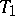, which can also be axiomatic. The method of interpretation can be used to establish relative consistency — i.e. to demonstrate statements of the type "if a theory T1 is consistent, so is the theory T" in the following manner. Assume that the theoryhas been interpreted in the theoryso that all axioms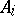ofare interpreted by true statements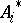of. All theorems of, i.e. any propositionwhich is logically deducible from the axiomsin, is interpreted inby some proposition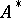, which is deducible infrom the interpretationsof the axioms, and is therefore true. This last statement is based on an additional implicit assumption to the effect that the logical tools of the theoriesandare to some extent similar, in practice this condition is usually satisfied. (In the initial applications of the method of interpretation, this assumption was not even discussed, since it was assumed to be self-evident; in fact, during the first attempts at demonstrating a relative consistency theorem, the logical tools ofandwere simply identical; this was the classical theory of predicates.) Now let the theorybe inconsistent, i.e. let both some statementof this theory and its negation be derivable in it. It then follows from the above that the propositionsand non-will both be true statements of, i.e.is inconsistent. This was the method employed by F. Klein and H. Poincaré to show that Lobachevskii's non-Euclidean geometry is consistent if Euclid's geometry is consistent; also, the problem of the consistency of Hilbert's axiomatization of Euclidean geometry was reduced by Hilbert to the problem of the consistency of arithmetic. The method of interpretation can also be used to solve the problem of independency of a systems of axioms: In order to prove that an axiomof a theoryis independent of the other axioms of that theory, i.e. cannot be deduced from them, and that its inclusion is therefore essential in order to obtain the complete theory, it is sufficient to construct an interpretation ofin whichis false, while all the other axioms are true. Another form of this manner of proving the independence ofis to establish the consistency of the theory obtained by replacinginby its negation. The result of the above-mentioned reduction of the problem of the consistency of Lobachevskii's geometry to the problem of the consistency of Euclidean geometry, and of reducing the problem of the consistency of Euclidean geometry to the problem of the consistency of arithmetic, is the conclusion that Euclid's fifth postulate is not derivable from the remaining geometrical axioms, provided that the arithmetic of positive integers is consistent. The weak point of the method of interpretation is the fact that, as far as problems of consistency and independence of axiom systems are concerned, it inevitably yields results of merely a relative character. However, its important achievement is the fact that it served to reveal that arithmetic plays a special role, in that it is a mathematical theory to whose consistency the problem of consistency of several other theories is reduced.

The method of the so-called formalism of the foundations of mathematics, due to Hilbert and his school, was a further step (and, in a sense, a peak) in the development of the method. It rendered the concept of an axiomatic theory more precise by introducing the notion of a formal system as the next stage in the development of the axiomatic method. As a result, it proved possible to treat the mathematical theories themselves as precise mathematical objects and to construct a general theory of such theories — a so-called meta-theory. The possibility of solving all the main problems in the foundations of mathematics in this way appeared very attractive, and Hilbert himself was tempted to follow this path. The principal concept in this approach was that of a formal system. Any formal system is constructed as a perfectly definite class of expressions — that is, formulas from which a subclass of formulas, known as the theorems of the formal system, is derived in a certain well-defined manner. The formulas of the formal system are not directly meaningful, and in general they may be constructed from signs or symbols merely chosen for reasons of technical convenience. In fact, the way of constructing formulas and the concept of a theorem of some formal system are so chosen that the resulting formal apparatus can be used for the most adequate expression of some mathematical (or even non-mathematical) theory, more precisely, as an expression of both its factual contents and its deductive structure. The general procedure for constructing an arbitrary formal systemis as follows.

I) The language of the system.

a) The alphabet is a list of elementary symbols of the system.

b) The construction (syntactic) rules are rules according to which the formulas ofare constructed from the elementary symbols. A sequence of elementary symbols is a taken as a formula if and only if it can be constructed by means of syntactic rules.

II) The axioms of the system. A certain set of formulas (usually a finite or countable set), said to be the axioms of the system, is specified.

III) The derivation rules of the system(or deduction rules). A (usually finite) population of predicates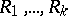is defined on the set of all formulas of. Let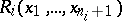be one of these predicates (); if, for given formulas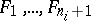, the statementis true, then one says that the formulafollows directly from the formulasby the rule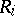.

Specifying I, II and III is the same as specifying the formal systemas a precise mathematical object, since the concept of a theorem or a derivable formula ofis formed for all formal systems in a uniform manner (the degree of accuracy depending on the level of accuracy of the alphabet, of the syntactic rules and of the derivation laws, i.e. of the predicates). A derivation inis any finite sequence of formulas in that system in which each formula is either an axiom ofor follows directly from some formulas which precede it in the sequence in accordance with one of the derivation lawsof. A formula ofis called a theorem of this system if there exists a derivation inending in this formula.

Any specific mathematical theorymay be translated into the language of a suitable formal systemso that any meaningful (true or false) statement ofcan be expressed by one of the formulas of.

It can naturally be expected that this method of formalization would make it possible to construct all the meaningful elements of any mathematical theory on the precise and apparently reliable basis represented by the concept of a derivable formula (a theorem of the formal system), and to solve fundamental problems such as the problem of the consistency of the mathematical theory by proving the corresponding statements about the formal system that formalizes this theory. Since the formal systems of the type just described are exact, or "finitistic" , using the term used by the school of Hilbert, mathematical objects, it could be expected that it would be possible to obtain finitistic proofs of consistency statements, i.e. proofs which would in a certain sense be effective, that is, independent of such powerful tools as, for example, the abstraction of actual infinity (which is one of the reasons for the difficulties encountered in the foundations of classical mathematical theories). Thus, the requirement that the tools employed in arriving at results concerning formal systems, and in particular concerning their consistency, be finitistic, represented a regular feature of Hilbert's formalistic program. However, the results obtained by K. Gödel in the early 1930s frustrated the main expectations based on this program. Gödel showed that:

1) Any natural consistent formalization of arithmetic or of any other mathematical theory which involves arithmetic (e.g. set theory) is incomplete and cannot be completed in the sense that a)contains (true with respect to their contents) unsolvable formulas, i.e. formulassuch that neithernor non-are derivable in(the incompleteness of formal arithmetic); b) whatever the finite set of supplementary axioms (e.g. of unsolvable formulas in) which are introduced to enlarge, the new, stronger, system thus produced inevitably contains its own unsolvable formulas (incompleteness; see  and Gödel incompleteness theorem).

2) If formal arithmetic is in fact consistent then, while the statement of its consistency is expressible in its own language, it is impossible to prove this statement by the methods of formalized arithmetic itself.

This means that, even in the case of arithmetic, it is intrinsically impossible to exhaust all its contents-wise true statements by a class of derivable formulas of any formal system, and that there is no hope of obtaining any finitistic proof of the consistency of arithmetic, since it seems that any reasonable precision of the concept of a finitistic proof is formalizable in formal arithmetic.

All this imposes definite limitations on the possibilities of the axiomatic method in the form it takes in the framework of Hilbert's formalism. However, within these limits it continues to play an important role in the foundations of mathematics. Thus, subsequent to the publication of Gödel's results, he himself (1938–1940) and P. Cohen (1963), basing themselves on the axiomatic approach with the use of the method of interpretation, obtained fundamental results concerning the compatibility and mutual independence of the axiom of choice and the continuum hypothesis in set theory , . As regards consistency, which is a fundamental problem in the foundations of mathematics, it had become clear in view of Gödel's results that it can be solved only with the aid of tools and ideas which are not finitistic. Various approaches to this question proved possible, not all of which are equally acceptable to mathematicians, mainly because of the differences of opinion as to the acceptability of various logical tools. Among the results obtained concerning the consistency of formal systems, one can mention, first of all, the proof of the consistency of formal arithmetic , based on infinite induction up to a certain countable transfinite number. Another, more recent, example of this kind is the attempt to use certain ideas of intuitionism for proving the consistency of the formal system of analysis .